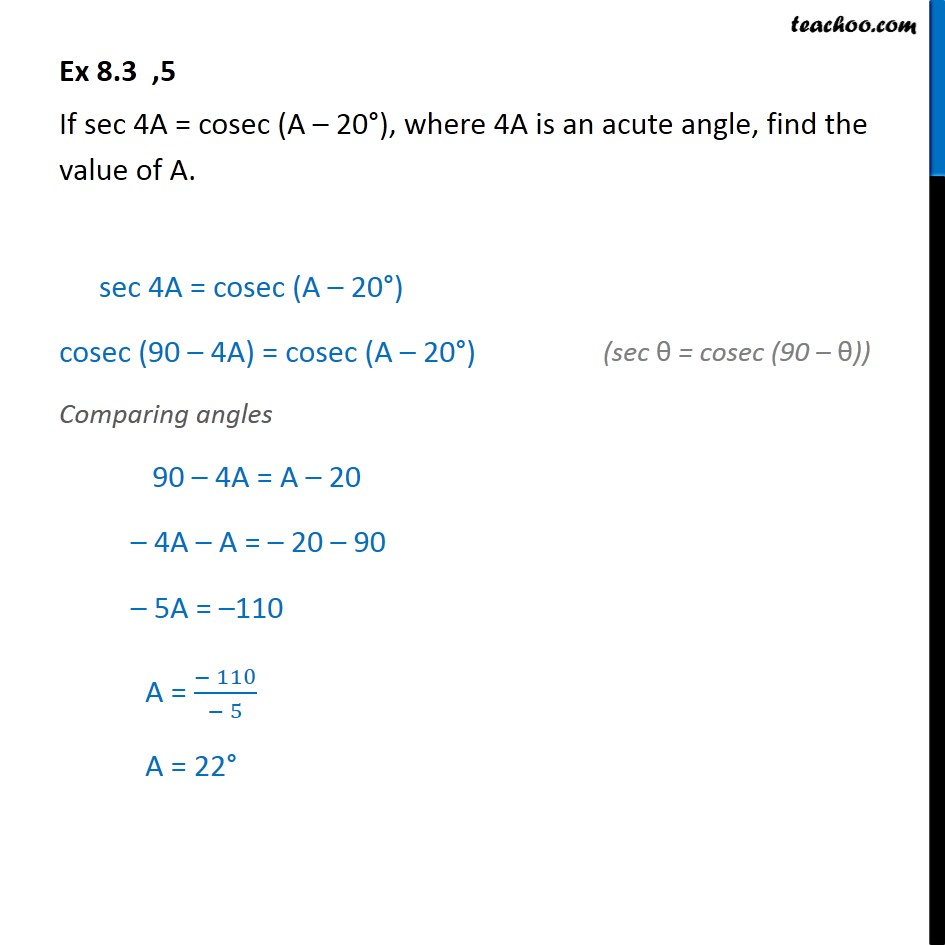Sin 90 - θ, cos 90 - θ formula

Chapter 8 Class 10 Introduction to Trignometry
Serial order wiseLearn in your speed, with individual attention - Teachoo Maths 1-on-1 Class

### Transcript

Ex 8.3 ,5 If sec 4A = cosec (A – 20°), where 4A is an acute angle, find the value of A. sec 4A = cosec (A – 20°) cosec (90 – 4A) = cosec (A – 20°) Comparing angles 90 – 4A = A – 20 – 4A – A = – 20 – 90 – 5A = –110 A = (− 110)/(− 5) A = 22°